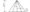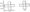# Engineering Graphics May 2008

Note: Attempt all questions. Part (a) is compulsory, attempt any two from part (b) (c) and (d).

Q1  (a). Fill up the blanks in the following sentences:

(i) The ratio of the length of the drawing of the object to the actual length of the object is called______ .

(ii) For drawing of small instruments, watches etc,_______ scale is always used.

(b)  A 10 mm ling line is represented by 15 cm draw a scale to measure up to 1 mm and show (1/100) of 25 mm.

(c) A ball is thrown in the air reaches a maximum height, of 45 meters and travels a horizontal distance of 75 meters, Trace the path of the ball assum ing it to be parabola (ue tangent method to draw)

(d) Draw a circle with diameter AB equal to 65 mm. Draw a line AC 150 mm long and tangent to the circle.Trace the path of A, when the line AC rolls on the circle without slippling.

Q2. (a) Draw symbols for First and Third angle methods of projection,

(b) Draw front view, top view and right side view of an object shown in fig-1.

(c) A point Q is 15 mm above H.P and lies in the first quadrent. Its shortest distance from xy is 40mm Draw its projections.

(d) A line AB, 75 mm long is in second quadrent with that end A in the H.P. and the end B in the V.P. The line is inclined at 30° to the HP and 45° to the VP Draw the projection ofAB and determine its traces.

Q3 (a) Draw only top view of an equailteral triangle of 3 cm side is lying on the ground with its one edge parallel to both the planes.

(b) The top view of a plate, the surface of which is perpendicular to the V.P.and inclined at 50° to the H.P. is a circle of 60 mm idameter. Draw its three views.

(c) A tetrahedran of 75 mm long edges has one edge parallel to the H.P. and  inclined at 45° to the V.P. while a face containing that edge is vertical. Draw its projection.

(d) A cone, diameter of the base 60 mm and axis 70 mm long is resting on its base on the H.P. It is cut by a section plane so that true shape of the section is parabola having 35mm base, and axis 60 mm. Draw the pain, the elevation and the true shape of the section.

Q4 (a) What are the methods of developments?

(b) Draw the development of the lateral surface of the part P of a pentagonal pyramid, one side of base parallel to the V.P. the. front view of which is shown in ‘fig-2(c) Draw the devel  of the lateral surface of the part p of a cube, one vertical face i,30° to the V.P., the front view of which is shown in fig-3.(d)Draw the isometric view of solid whose orthographic projection are given in fig-4.Q5 (a) Write four names of CAD softwares

(b) Write commands to draw line by:

(i) Using absolute co-ordinates,

(ii) Using relative co-ordinates,

(iii) Using polar co-ordinates,

(c) What is concept and utility of layer?

(d) Write commands for:

(i)Rectangular array

(ii)Rectangle

(iii)Extrude

(iv)Pline